Documentation

This is machine translation

Mouseover text to see original. Click the button below to return to the English version of the page.

interp2

Interpolation for 2-D gridded data in meshgrid format

Syntax

``Vq = interp2(X,Y,V,Xq,Yq)``
``Vq = interp2(V,Xq,Yq)``
``Vq = interp2(V)``
``Vq = interp2(V,k)``
``Vq = interp2(___,method)``
``Vq = interp2(___,method,extrapval)``

Description

example

````Vq = interp2(X,Y,V,Xq,Yq)` returns interpolated values of a function of two variables at specific query points using linear interpolation. The results always pass through the original sampling of the function. `X` and `Y` contain the coordinates of the sample points. `V` contains the corresponding function values at each sample point. `Xq` and `Yq` contain the coordinates of the query points.```
````Vq = interp2(V,Xq,Yq)` assumes a default grid of sample points. The default grid points cover the rectangular region, `X=1:n` and `Y=1:m`, where `[m,n] = size(V)`. Use this syntax when you want to conserve memory and are not concerned about the absolute distances between points.```
````Vq = interp2(V)` returns the interpolated values on a refined grid formed by dividing the interval between sample values once in each dimension.```

example

````Vq = interp2(V,k)` returns the interpolated values on a refined grid formed by repeatedly halving the intervals `k` times in each dimension. This results in `2^k-1` interpolated points between sample values.```

example

````Vq = interp2(___,method)` specifies an alternative interpolation method: `'linear'`, `'nearest'`, `'cubic'`, `'makima'`, or `'spline'`. The default method is `'linear'`.```

example

````Vq = interp2(___,method,extrapval)` also specifies `extrapval`, a scalar value that is assigned to all queries that lie outside the domain of the sample points. If you omit the `extrapval` argument for queries outside the domain of the sample points, then based on the `method` argument `interp2` returns one of the following: Extrapolated values for the `'spline'` and `'makima'` methods`NaN` values for other interpolation methods ```

Examples

collapse all

Coarsely sample the `peaks` function.

```[X,Y] = meshgrid(-3:3); V = peaks(X,Y);```

Plot the coarse sampling.

```figure surf(X,Y,V) title('Original Sampling');```Create the query grid with spacing of 0.25.

`[Xq,Yq] = meshgrid(-3:0.25:3);`

Interpolate at the query points.

`Vq = interp2(X,Y,V,Xq,Yq);`

Plot the result.

```figure surf(Xq,Yq,Vq); title('Linear Interpolation Using Finer Grid');```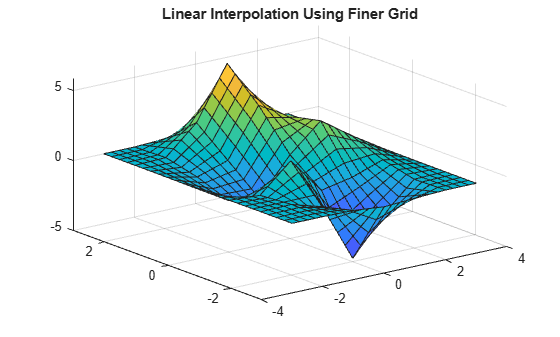Coarsely sample the peaks function.

```[X,Y] = meshgrid(-3:3); V = peaks(7);```

Plot the coarse sampling.

```figure surf(X,Y,V) title('Original Sampling');```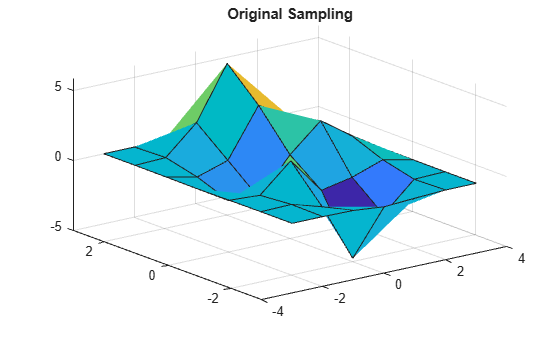Create the query grid with spacing of 0.25.

`[Xq,Yq] = meshgrid(-3:0.25:3);`

Interpolate at the query points, and specify cubic interpolation.

`Vq = interp2(X,Y,V,Xq,Yq,'cubic');`

Plot the result.

```figure surf(Xq,Yq,Vq); title('Cubic Interpolation Over Finer Grid');```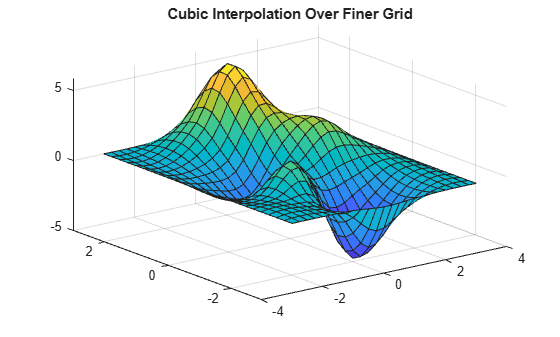Load some image data into the workspace.

`load clown`

Isolate a small region of the image and cast it to single.

`V = single(X(1:124,75:225));`

Display the image.

```figure imagesc(V); colormap gray axis image axis off title('Original Image');```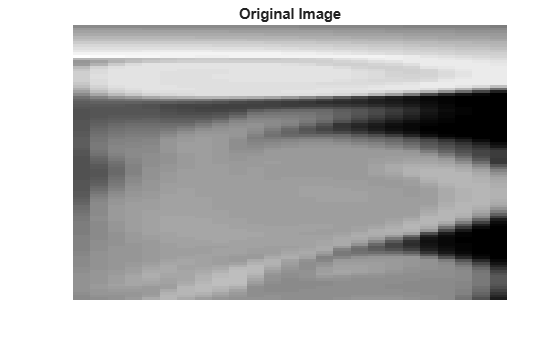Insert interpolated values by repeatedly dividing the intervals between points of the refined grid five times in each dimension.

`Vq = interp2(V,5);`

Display the result.

```figure imagesc(Vq); colormap gray axis image axis off title('Linear Interpolation');```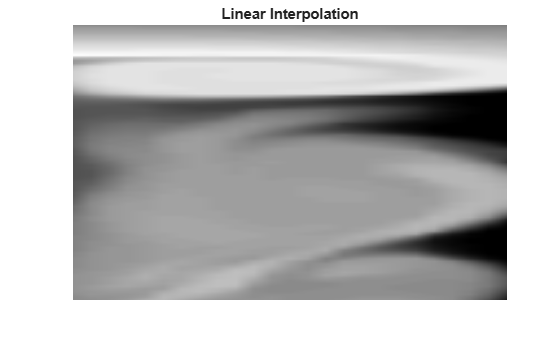Coarsely sample a function over the range, `[-2, 2]` in both dimensions.

```[X,Y] = meshgrid(-2:0.75:2); R = sqrt(X.^2 + Y.^2)+ eps; V = sin(R)./(R);```

Plot the coarse sampling.

```figure surf(X,Y,V) xlim([-4 4]) ylim([-4 4]) title('Original Sampling')```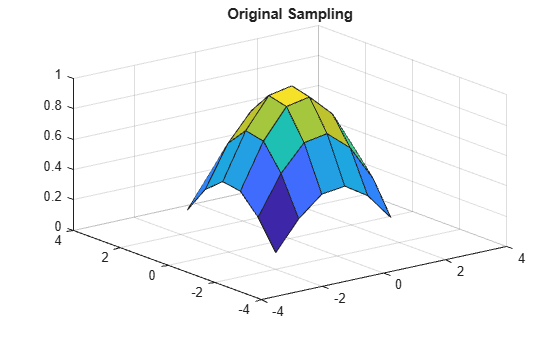Create the query grid that extends beyond the domain of `X` and `Y`.

`[Xq,Yq] = meshgrid(-3:0.2:3);`

Perform cubic interpolation within the domain of `X` and `Y`, and assign all queries that fall outside to zero.

`Vq = interp2(X,Y,V,Xq,Yq,'cubic',0);`

Plot the result.

```figure surf(Xq,Yq,Vq) title('Cubic Interpolation with Vq=0 Outside Domain of X and Y');```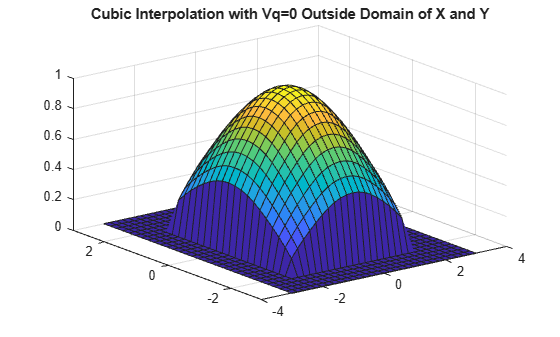Input Arguments

collapse all

Sample grid points, specified as real matrices or vectors. The sample grid points must be unique.

• If `X` and `Y` are matrices, then they contain the coordinates of a full grid (in meshgrid format). Use the `meshgrid` function to create the `X` and `Y` matrices together. Both matrices must be the same size.

• If `X` and `Y` are vectors, then they are treated as a grid vectors. The values in both vectors must be strictly monotonic and increasing.

Note

In a future release, `interp2` will not accept mixed combinations of row and column vectors for the sample and query grids. Instead, you must construct the full grid using `meshgrid`. Alternatively, if you have a large data set, you can use `griddedInterpolant` instead of `interp2`.

Example: `[X,Y] = meshgrid(1:30,-10:10)`

Data Types: `single` | `double`

Sample values, specified as a real or complex matrix. The size requirements for `V` depend on the size of `X` and `Y`:

• If `X` and `Y` are matrices representing a full grid (in `meshgrid` format), then `V` must be the same size as `X` and `Y`.

• If `X` and `Y` are grid vectors, then `V` must be a matrix containing `length(Y)` rows and `length(X)` columns.

If `V` contains complex numbers, then `interp2` interpolates the real and imaginary parts separately.

Example: `rand(10,10)`

Data Types: `single` | `double`
Complex Number Support: Yes

Query points, specified as a real scalars, vectors, matrices, or arrays.

• If `Xq` and `Yq` are scalars, then they are the coordinates of a single query point.

• If `Xq` and `Yq` are vectors of different orientations, then `Xq` and `Yq` are treated as grid vectors.

• If `Xq` and `Yq` are vectors of the same size and orientation, then `Xq` and `Yq` are treated as scattered points in 2-D space.

• If `Xq` and `Yq` are matrices, then they represent either a full grid of query points (in `meshgrid` format) or scattered points.

• If `Xq` and `Yq` are N-D arrays, then they represent scattered points in 2-D space.

Note

In a future release, `interp2` will not accept mixed combinations of row and column vectors for the sample and query grids. Instead, you must construct the full grid using `meshgrid`. Alternatively, if you have a large data set, you can use `griddedInterpolant` instead of `interp2`.

Example: `[Xq,Yq] = meshgrid((1:0.1:10),(-5:0.1:0))`

Data Types: `single` | `double`

Refinement factor, specified as a real, nonnegative, integer scalar. This value specifies the number of times to repeatedly divide the intervals of the refined grid in each dimension. This results in `2^k-1` interpolated points between sample values.

If `k` is `0`, then `Vq` is the same as `V`.

`interp2(V,1)` is the same as `interp2(V)`.

The following illustration shows the placement of interpolated values (in red) among nine sample values (in black) for `k=2`.Example: `interp2(V,2)`

Data Types: `single` | `double`

Interpolation method, specified as one of the options in this table.

`'linear'`The interpolated value at a query point is based on linear interpolation of the values at neighboring grid points in each respective dimension. This is the default interpolation method.C0
• Requires at least two grid points in each dimension

• Requires more memory than `'nearest'`

`'nearest'`The interpolated value at a query point is the value at the nearest sample grid point. Discontinuous
• Requires two grid points in each dimension.

• Fastest computation with modest memory requirements

`'cubic'`The interpolated value at a query point is based on a cubic interpolation of the values at neighboring grid points in each respective dimension. The interpolation is based on a cubic convolution.C1
• Grid must have uniform spacing in each dimension, but the spacing does not have to be the same for all dimensions

• Requires at least four points in each dimension

• Requires more memory and computation time than `'linear'`

`'makima'`Modified Akima cubic Hermite interpolation. The interpolated value at a query point is based on a piecewise function of polynomials with degree at most three evaluated using the values of neighboring grid points in each respective dimension. The Akima formula is modified to avoid overshoots.C1
• Requires at least 2 points in each dimension

• Produces fewer undulations than `'spline'`

• Computation time is typically less than `'spline'`, but the memory requirements are similar

`'spline'`The interpolated value at a query point is based on a cubic interpolation of the values at neighboring grid points in each respective dimension. The interpolation is based on a cubic spline using not-a-knot end conditions.C2
• Requires four points in each dimension

• Requires more memory and computation time than `'cubic'`

Function value outside domain of `X` and `Y`, specified as a real or complex scalar. `interp2` returns this constant value for all points outside the domain of `X` and `Y`.

Example: `5`

Example: `5+1i`

Data Types: `single` | `double`
Complex Number Support: Yes

Output Arguments

collapse all

Interpolated values, returned as a real or complex scalar, vector, or matrix. The size and shape of `Vq` depends on the syntax you use and, in some cases, the size and value of the input arguments.

SyntaxesSpecial ConditionsSize of VqExample
`interp2(X,Y,V,Xq,Yq)`
`interp2(V,Xq,Yq)`
and variations of these syntaxes that include `method` or `extrapval`
`Xq`, `Yq` are scalarsScalar`size(Vq) = [1 1]` when you pass `Xq` and `Yq` as scalars.
Same as above`Xq`, `Yq` are vectors of the same size and orientationVector of same size and orientation as `Xq` and `Yq`If `size(Xq) = [100 1]`
and ```size(Yq) = [100 1]```,
then ```size(Vq) = [100 1]```.
Same as above`Xq`, `Yq` are vectors of mixed orientationMatrix in which the number of rows is `length(Yq)`, and the number of columns is `length(Xq)`If `size(Xq) = [1 100]`
and ```size(Yq) = [50 1]```,
then `size(Vq) = [50 100]`.
Same as above`Xq`, `Yq` are matrices or arrays of the same sizeMatrix or array of the same size as `Xq` and `Yq`If `size(Xq) = [50 25]`
and ```size(Yq) = [50 25]```,
then ```size(Vq) = [50 25]```.
`interp2(V,k)`
and variations of this syntax that include `method` or `extrapval`
None

Matrix in which the number of rows is:
```2^k * (size(V,1)-1)+1```,

and the number of columns is:
`2^k * (size(V,2)-1)+1`

If `size(V) = [10 20]`
and ```k = 2```,
then `size(Vq) = [37 77]`.

collapse all

Strictly Monotonic

A set of values that are always increasing or decreasing, without reversals. For example, the sequence, ```a = [2 4 6 8]``` is strictly monotonic and increasing. The sequence, ```b = [2 4 4 6 8]``` is not strictly monotonic because there is no change in value between `b(2)` and `b(3)`. The sequence, `c = [2 4 6 8 6]` contains a reversal between `c(4)` and `c(5)`, so it is not monotonic at all.

Full Grid (in meshgrid Format)

For `interp2`, the full grid is a pair of matrices whose elements represent a grid of points over a rectangular region. One matrix contains the x-coordinates, and the other matrix contains the y-coordinates. The values in the x-matrix are strictly monotonic and increasing along the rows. The values along its columns are constant. The values in the y-matrix are strictly monotonic and increasing along the columns. The values along its rows are constant. Use the `meshgrid` function to create a full grid that you can pass to `interp2`.

For example, the following code creates a full grid for the region, –1 ≤ x ≤ 3 and 1 ≤ y ≤ 4:

`[X,Y] = meshgrid(-1:3,(1:4))`
```X = -1 0 1 2 3 -1 0 1 2 3 -1 0 1 2 3 -1 0 1 2 3 Y = 1 1 1 1 1 2 2 2 2 2 3 3 3 3 3 4 4 4 4 4```

Grid vectors are a more compact format to represent a grid than the full grid. The relation between the two formats and the matrix of sample values `V` is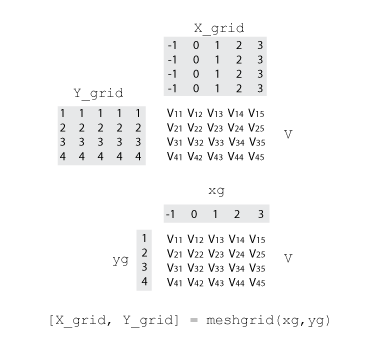Grid Vectors

For `interp2`, grid vectors consist of a pair of vectors that define the x- and y-coordinates in a grid. The row vector defines x-coordinates, and the column vector defines y-coordinates.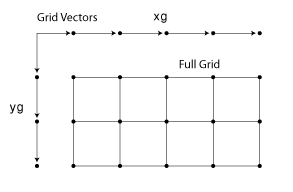For example, the following code creates the grid vectors that specify the region, –1 ≤ x ≤ 3 and 1 ≤ y ≤ 4:

```x = -1:3; y = (1:4)';```

Scattered Points

For `interp2`, scattered points consist of a pair of arrays that define a collection of points scattered in 2-D space. One array contains the x-coordinates, and the other contains the y-coordinates.

For example, the following code specifies the points, (2,7), (5,3), (4,1), and (10,9):

```x = [2 5; 4 10]; y = [7 3; 1 9];```